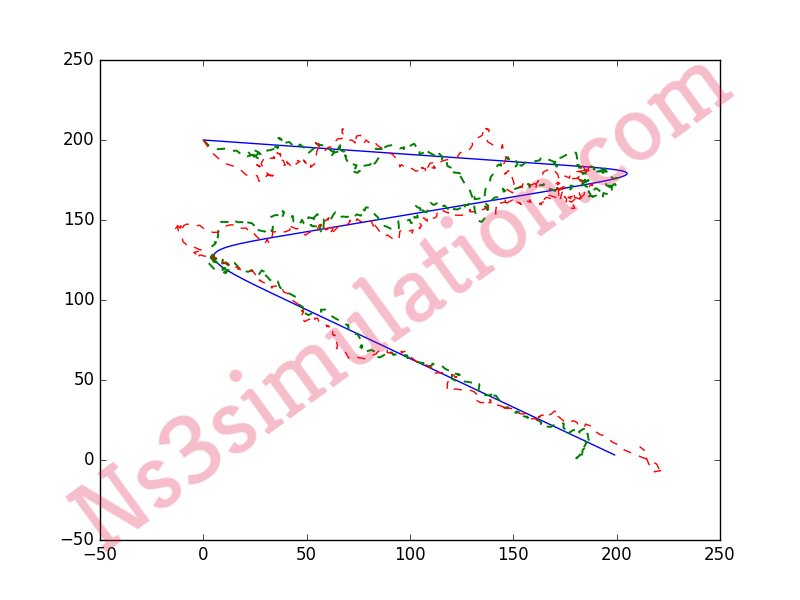Ns3 Projects for B.E/B.Tech M.E/M.Tech PhD Scholars.  Phone-Number:9790238391   E-mail: ns3simulation@gmail.com

# Wireless Sensor Networks Projects with Source Code in Python

As the matter of fact, our experienced technical professionals are well qualified and ready to provide appropriate guidance. Without any delay, let’s see the list of titles for the WSN research projects using python in the following.

• Online indoor localization using DOA of wireless signals
• Statistical method based fault detection algorithm for wireless sensor networks
• Localization system in wireless sensor networks
• Vehicle to vehicle communication system using IoT

Next, we have highlighted the sample source code based on python for the trajectory process among the nodes.

``` import numpy as np import matplotlib.pyplot as plt def assemble(X2,Y2): pos=np.zeros([len(X2),2]) for i in range(len(X2)): pos[i]=np.matrix([[X2[i],Y2[i]]]) return pos X1 = np.arange(50,150,1) Y1 = np.arange(50,150,1) tra1=assemble(X1,Y1) X2=np.arange(0,200,1) Y2=-np.exp(0.0266*X2) +200 tra2 = assemble(X2,Y2) x1 = np.arange(0,220,1) y1 = np.arange(200,180,-.09) x2 = np.arange(220,-10,-1) y2 = np.arange(179,129,-.2173) dy = .5 x3 = np.arange(-10,200,1) y3 = np.arange(128,0,-.609) x1= np.append(x1,x2) x = np.append(x1,x3) y1 = np.append(y1,y2) y = np.append(y1,y3) path = np.zeros((len(x),2)) for i in range(len(x)): path[i] = np.matrix([x[i],y[i]]) def smooth(path,data_weight=0.3 ,smooth_weight=0.15, tolerance = 0.01): newpath = path change = tolerance c =0 while c < 1000 : change =0. for i in range(1,len(path)-1): if not(i ==99 or i==100 or i==199 or i==200 or i==299): for j in range(len(path)): aux = newpath[i][j] newpath[i][j] += data_weight * (path[i][j]-newpath[i][j]) newpath[i][j] += smooth_weight * ( newpath[i-1][j] + newpath[i+1][j] - 2.*newpath[i][j] ) change += abs(aux - newpath[i][j]) else : for j in range(len(path)): aux = newpath[i][j] newpath[i][j] += 0.8 * (path[i][j]-newpath[i][j]) newpath[i][j] += 0.1 * ( newpath[i-1][j] + newpath[i+1][j] - 2.*newpath[i][j] ) change += abs(aux - newpath[i][j]) change += 0.005 c+=1 return newpath tra3 = smooth(path) #np.save('tra1.npy',tra1) plt.plot(tra1[:,0],tra1[:,1]) plt.show() plt.plot(tra2[:,0],tra2[:,1]) plt.show() plt.plot(tra3[:,0],tra3[:,1]) plt.show() ```

Then, we have to implement the wireless sensor network in python through going to the menu and click the run module option.At last, we have highlighted the result which is obtained through execution of wireless sensor network in python.Don’t worry if you guys have any doubts, because our technical experts are always ready to help you out.